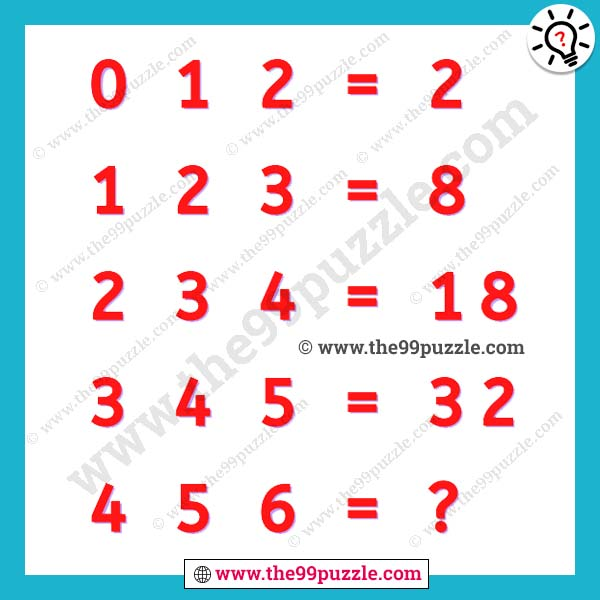# Math puzzle question for kids with answer – Puzz261

This math puzzle is very fun and tricky. If you think out of the box you can solve this quickly. This math puzzle question for kids. Here this math problem you see some math equations, you have to find out the math trick and solve the last equation answer. Challenge yourself with different levels of math puzzles and stretch the limits of your intelligence. Math puzzles and math riddles can be a great source of learning and provide a better understanding to the kids.

0 1 2 = 2

1 2 3 = 8

2 3 4 = 18

3 4 5 = 32

4 5 6 = ?###### Explanation:

0 1 2 = (0×1)+(1×2) = 2

1 2 3 = (1×2)+(2×3) = 8

2 3 4 = (2×3)+(3×4) = 18

3 4 5 = (3×4)+(4×5) = 32

4 5 6 = (4×5)+(5×6) = 50